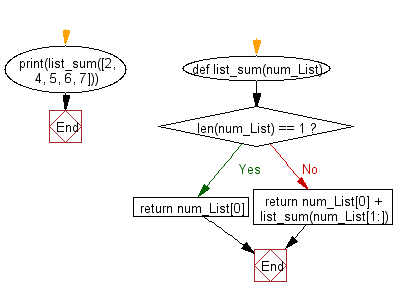﻿ Python Data Structures and Algorithms - Recursion: Binary search - w3resource# Python Data Structures and Algorithms - Recursion: Sum of a list of numbers

## Python Recursion: Exercise-1 with Solution

Write a Python program to calculate the sum of a list of numbers.

Sample Solution:

Python Code:

``````def list_sum(num_List):
if len(num_List) == 1:
return num_List
else:
return num_List + list_sum(num_List[1:])

print(list_sum([2, 4, 5, 6, 7]))
```
```

Sample Output:

```24
```

Flowchart:## Visualize Python code execution:

The following tool visualize what the computer is doing step-by-step as it executes the said program:

Python Code Editor:

What is the difficulty level of this exercise?

Test your Programming skills with w3resource's quiz.

﻿

## Python: Tips of the Day

Decapitalizes the first letter of a string:

Example:

```def tips_decapitalize(s, upper_rest=False):
return s[:1].lower() + (s[1:].upper() if upper_rest else s[1:])
print(tips_decapitalize('PythonTips'))
print(tips_decapitalize('PythonTips', True))
```

Output:

```pythonTips
pYTHONTIPS
```